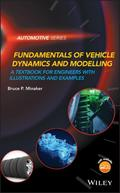John Wiley & Sons Fundamentals of Vehicle Dynamics and ModellingAn introduction to vehicle dynamics and the fundamentals of mathematical modeling Fundamentals of V.. Product #: 978-1-118-98009-5 Regular price: \$85.89 \$85.89 Auf Lager

# Fundamentals of Vehicle Dynamics and Modelling

## A Textbook for Engineers With Illustrations and Examples

Automotive Series1. Auflage September 2019
300 Seiten, Hardcover
Wiley & Sons Ltd

ISBN: 978-1-118-98009-5
John Wiley & Sons

Jetzt kaufen

### Preis: 91,90 €

Preis inkl. MwSt, zzgl. Versand

An introduction to vehicle dynamics and the fundamentals of mathematical modeling

Fundamentals of Vehicle Dynamics and Modeling is a student-focused textbook providing an introduction to vehicle dynamics, and covers the fundamentals of vehicle model development. It illustrates the process for construction of a mathematical model through the application of the equations of motion. The text describes techniques for solution of the model, and demonstrates how to conduct an analysis and interpret the results. A significant portion of the book is devoted to the classical linear dynamic models, and provides a foundation for understanding and predicting vehicle behaviour as a consequence of the design parameters.

Modeling the pneumatic tire is also covered, along with methods for solving the suspension kinematics problem, and prediction of acceleration and braking performance. The book introduces the concept of multibody dynamics as applied to vehicles and provides insight into how large and high fidelity models can be constructed. It includes the development of a method suitable for computer implementation, which can automatically generate and solve the linear equations of motion for large complex models.

Key features:

* Accompanied by a website hosting MATLAB® code.

* Supported by the Global Education Delivery channels.

Fundamentals of Vehicle Dynamics and Modeling is an ideal textbook for senior undergraduate and graduate courses on vehicle dynamics.

Dedication

Preface

Acknowledgement

List of Symbols xv

1 Introduction 1

1.1 Past, present, and future 2

References 4

2 Tire Modelling 5

2.1 Rolling losses 5

2.2 Longitudinal force 7

2.3 Lateral force 9

2.4 Vertical moments 11

2.5 Normal force 12

References 14

3 Longitudinal Dynamics 15

3.1 Acceleration performance 16

3.1.1 Engine limited performance 16

3.1.2 Tire limited acceleration 21

3.2 Braking performance 23

4 Linear Dynamic Models 31

4.1 .e yaw plane model 31

4.1.2 Transient analysis 42

4.1.3 Frequency response 48

4.1.4 Time history solution 52

4.2 .e truck and trailer model 53

4.2.2 Transient analysis 57

4.3 .e quarter car model 60

4.3.1 Transient analysis 63

4.3.2 Frequency response 68

4.4 .e bounce-pitch model 73

4.4.1 Transient analysis 75

4.4.2 Frequency response 82

References 91

5 Full Car Model 93

5.1.1 .e bounce-pitch-roll model 94

5.1.2 .e lateral-yaw model 97

5.2 Transient analysis 99

5.3 Kinematic effects 101

5.3.1 Roll centres 103

5.3.2 Quasi static model, with roll centres 106

5.4 Numerical solution of suspension kinematics 110

5.4.1 Algebraic equations formulation 110

5.4.2 Differential equations formulation 112

5.4.3 Tire orientation effects 114

5.5 Suspension damping 116

References 119

6 Multibody Dynamics 121

6.1 Generating the governing equations 121

6.1.1 Preliminary definitions 122

6.2 Definition of coordinates 123

6.3 Kinematic differential equations 125

6.4 Newton Euler equations 128

6.4.1 Inertial forces 129

6.4.2 Elastic forces 130

6.4.3 Linear spring 132

6.5 Constraint equations 137

6.5.1 Spherical joint 139

6.6 State space form 141

6.7 Example systems 144

6.7.1 Rigid rider bicycle 144

6.7.2 Multibody quarter car 150

References 155

7 Mathematics 157

7.1 Algebraic equations 157

7.1.1 Nonlinear algebraic equations 157

7.1.2 Linear algebraic equations 158

7.2 Differential equations 159

7.2.1 Nonlinear differential equations 160

7.2.2 Linear first order ODEs 160

7.2.3 Eigen analysis 161

7.2.4 Linear second order ODEs 164

7.2.5 Frequency response analysis 167

7.3 Differential algebraic equations 172

7.3.1 Differential equation approach 172

7.3.2 Algebraic equation approach 173

7.3.3 Linear differential algebraic equations 174

A Appendix A 177

A.1 Algebraic equations 177

A.2 Differential equations 178

Index 183
Dr Bruce P. Minaker is an Associate Professor in the Department of Mechanical, Automotive, & Materials Engineering at the University of Windsor. His primary research interest is in modeling, simulation, and control of vehicle dynamics. For several years he has been developing software to automatically generate the equations of motion of multibody systems, particularly for use in modeling vehicle dynamics. A strong proponent of open source software; he makes all the code freely available for download. He teaches a number of courses in related areas, including the senior vehicle design projects. Dr Minaker earned his graduate degrees at Queen's University and his undergraduate degree at the University of Waterloo.